# How to draw stop loss and take profit in trading viewThis feature lets you estimate how an order will go if you go long or short, shows the Profit & Loss (PnL) and estimates risk and closing account balance when price reaches your profit target or stop loss levels.

You can practice placing orders and seeing results without actually having to place the orders — get confident and knowledgeable before risking real money in the markets.

#### Calculate your Position Size and Account Balance

1. Create a Long Position or Short Position drawing.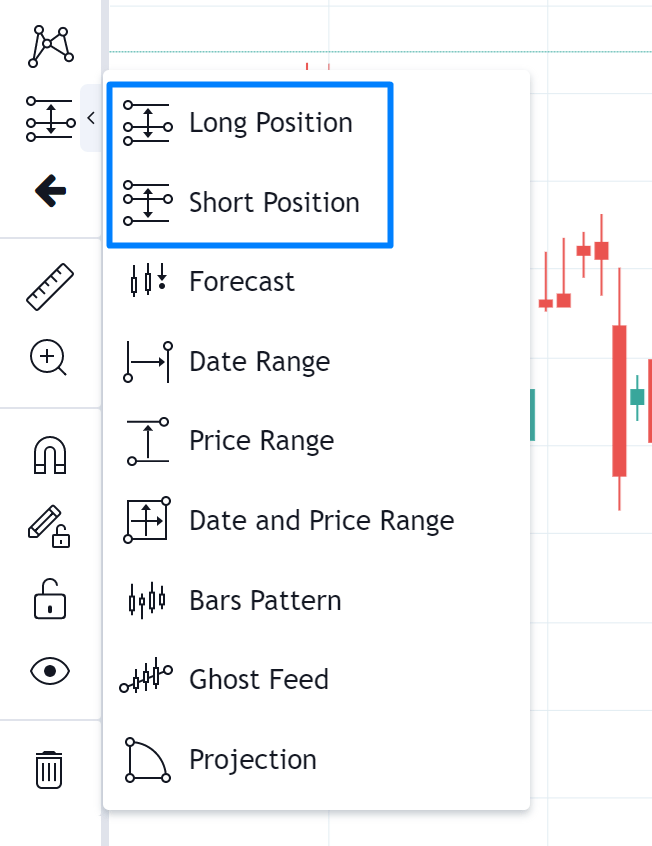2. In properties dialog of the instrument enter your initial account size and risk amount (either in absolute numbers or as a % of your account size). You can also enter the Lot Size. Click OK to accept.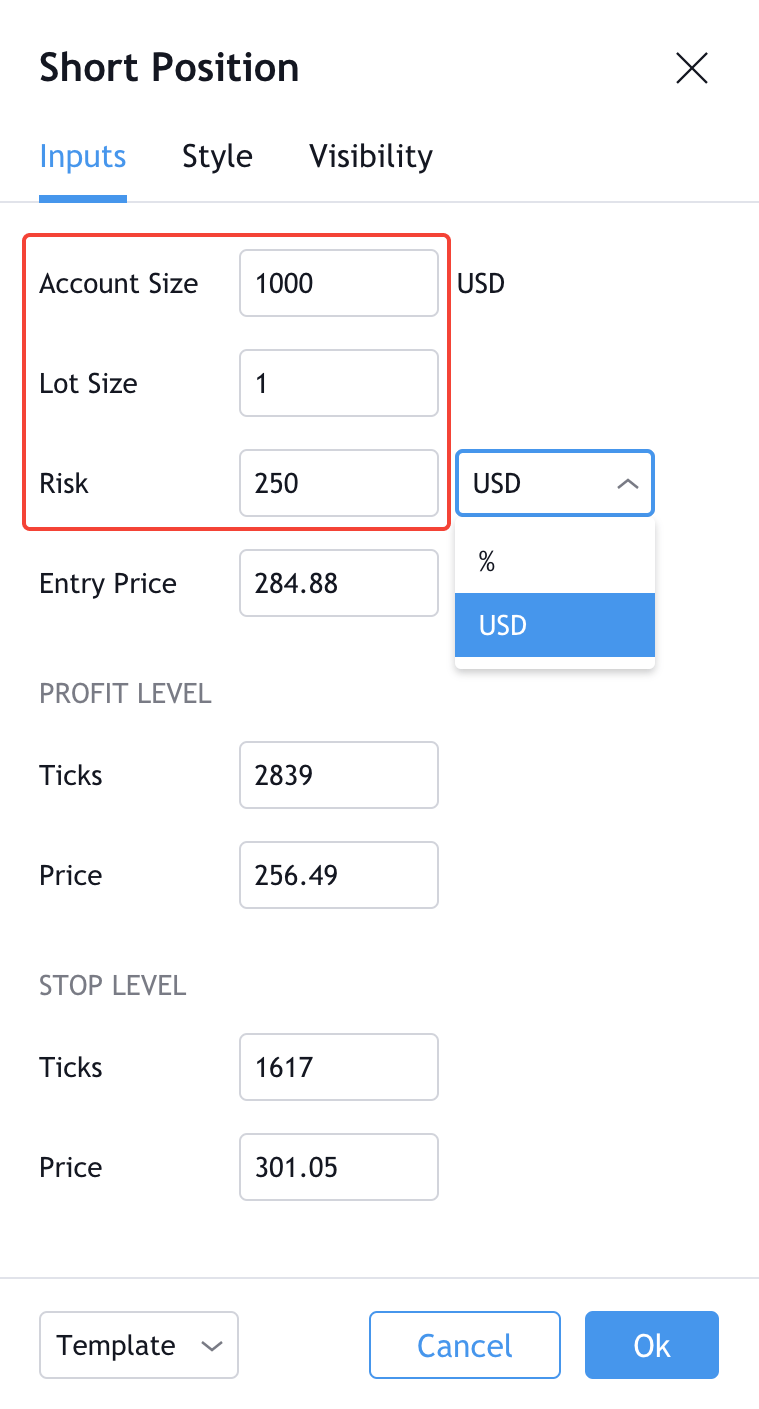3. Drawing tool tags will show you position size (1) and account balance when positions are closed after reaching either the Take Profit (2) or the Stop Loss (3) level.

#### How are the Position Size and Account Balance calculated?

##### Long Position:

Position Size

Qty = (RiskSize / ((EntryPrice – StopPrice) * Point value)) / Lot Size

Account Balance when position is closed after reaching the Take Profit level

Amount = AccountSize + (ProfitLevel – EntryPrice) * Qty * Point value

Account Balance when position is closed after reaching the Stop Loss level

Amount = AccountSize — (EntryPrice – StopLevel) * Qty *  Point value

##### Short Position:

Position Size

Qty = (RiskSize / ((StopPrice – EntryPrice) * Point Value)) / Lot Size

Account Balance when position is closed after reaching the Take Profit level

Amount = AccountSize + (EntryPrice – ProfitLevel) * Qty *  Point value

Account Balance when position is closed after reaching the Stop Loss level

Amount = AccountSize — (StopLevel – EntryPrice) * Qty * Point value

• AccountSize — initial account size specified in the settings
• RiskSize =
• Risk, if risk is selected in absolute numbers,
• Risk / 100 * AccountSize, if risk is selected as a percentage of account size.

#### Display performance in the Compact Mode

Try using the Compact Mode if you want the position performance tags to consume less space on the chart. You can enable by choosing the Compact checkbox in Settings.The new Long & Short Position drawing tool is here! This highly demanded feature lets you estimate how an order will go if you go long or short, shows the Profit & Loss (PnL) and estimates risk and closing account balance when price reaches your profit target or stop loss levels.

Now you can practice placing orders and seeing results without actually having to place the orders – get confident and knowledgeable before risking real money in the markets.

## Calculate your Position Size and Account Balance

1.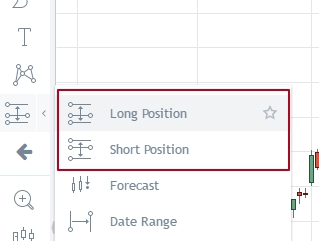Create a Long Position or Short Position drawing.

1.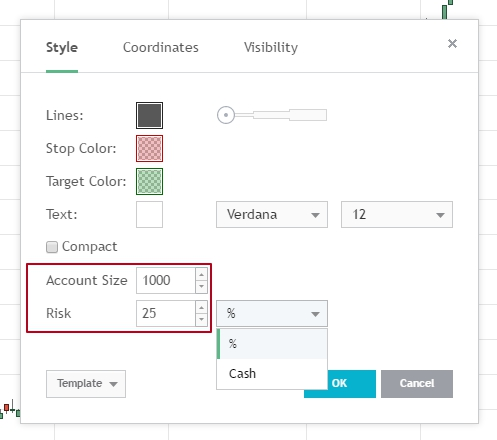Enter your initial account size and risk amount (either in absolute numbers or as a % of your account size), and click OK to accept.

Drawing tool tags will show you position size (1) and account balance when positions are closed after reaching either the Take Profit (2) or the Stop Loss (3) level.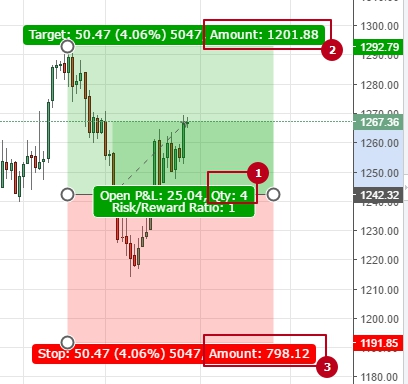How are the Position Size and Account Balance calculated?

Long Position

Position Size

Qty = RiskSize / (EntryPrice – StopPrice)

Account Balance when position is closed after reaching the Take Profit level

Amount = AccountSize + (ProfitLevel – EntryPrice) * Qty

Account Balance when position is closed after reaching the Stop Loss level

Amount = AccountSize – (EntryPrice – StopLevel) * Qty

Short Position

Position Size

Qty = RiskSize / (StopPrice – EntryPrice)

Account Balance when position is closed after reaching the Take Profit level

Amount = AccountSize + (EntryPrice – ProfitLevel) * Qty

Account Balance when position is closed after reaching the Stop Loss level

Amount = AccountSize – (StopLevel – EntryPrice) * Qty

• AccountSize – initial account size specified in the settings

• RiskSize

• =

Risk, if risk is selected in absolute numbers,

• = Risk / 100 * AccountSize, if risk is selected as a percentage of account size.

## Display performance in the Compact Mode

Try using the Compact Mode if you want the position performance tags to consume less space on the chart. You can enable by choosing the Compact checkbox in Settings.## Plotting Stop Losses and Take Profits

In Pine Script send stop loss and take profit orders by using the `stop`and`limit` parameters for the `strategy.exit()` and `strategy.order()`functions. In fact, the `strategy.entry()`function also supports sending `stop`and`limit`orders. However, that would be considered an entry rather than a stop loss or take profit and thus out of the scope of this article. Having said that, the techniques used in this article can be used for entries and just about any line you want to track/plot.

### Sending Orders

There are a couple of the reasons why people can sometimes find plotting the stop loss and take profit lines a little challenging:

1. They are using the `profit`and`loss`parameters instead of `stop`and`limit`
2. They calculate the stop loss and/or profit level once at the time of entry.

The `profit`and `loss` are measured in `ticks`. As such, the numbers we use to feed these parameters do not provide a natural price level we can plot. As such, a bit more work would be required to plot the level. Note: For more information on the various stop-loss options, `stop` of a long entry at `low``ATR` (`strategy.exit()` function inside an `if`statement, you can find that the stop line bounces around every bar as the `ATR`value is updated.

### Example

Before we move into a solution, let’s run through a quick example of placing `strategy.exit()`inside an `if`statement in case you are having trouble visualizing it. The example below will calculate the `stop` level and only send the order once when a `longCondition`or`shortCondition`is met.

`//@version=3strategy("My Strategy", overlay=true)ATR = atr(7)longCondition = crossover(sma(close, 100), sma(close, 200))if (longCondition) stop_level = low - ATR profit_level = close + ATR strategy.entry("My Long Entry Id", strategy.long) strategy.exit("TP/SL", "My Long Entry Id", stop=stop_level, limit=profit_level)shortCondition = crossunder(sma(close, 100), sma(close, 200))if (shortCondition) stop_level = high + ATR profit_level = close - ATR strategy.entry("My Short Entry Id", strategy.short) strategy.exit("TP/SL", "My Short Entry Id", stop=stop_level, limit=profit_level)`

This will result in a chart that looks something like this: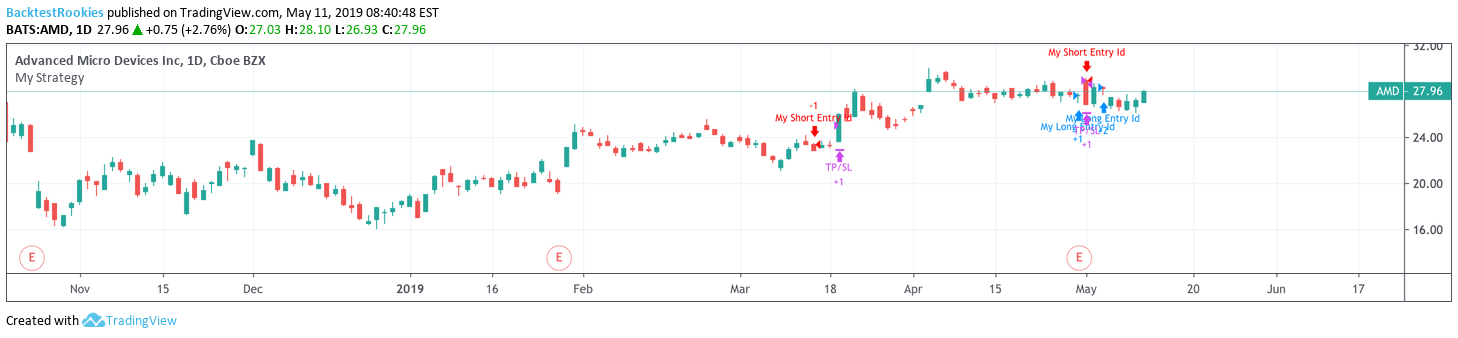## Plotting Solution

The key to plotting stop losses and take profits is to create a `series` (new line/variable) that is updated only when your stop-loss is updated. For example, if you only send a stop loss order once when the `longCondition`is met, then we similarly we only update our new `series` at that time too. Otherwise, we keep the same value as the previous bar. We can do this using something called a

`//@version=3strategy("My Strategy", overlay=true)ATR = atr(7)longCondition = crossover(sma(close, 100), sma(close, 200))if (longCondition) strategy.entry("My Long Entry Id", strategy.long) shortCondition = crossunder(sma(close, 100), sma(close, 200))if (shortCondition) strategy.entry("My Short Entry Id", strategy.short) long_stop_level = nalong_profit_level = nalong_stop_level := longCondition ? low - ATR : long_stop_levellong_profit_level := longCondition ? close + ATR : long_profit_levelshort_stop_level = nashort_profit_level = nashort_stop_level := shortCondition ? high + ATR : short_stop_levelshort_profit_level := shortCondition ? close - ATR : short_profit_levelstrategy.exit("TP/SL", "My Long Entry Id", stop=long_stop_level, limit=long_profit_level) strategy.exit("TP/SL", "My Short Entry Id", stop=short_stop_level, limit=short_profit_level) plot(strategy.position_size <= 0 ? na : long_stop_level, color=red, style=linebr, linewidth=2)plot(strategy.position_size <= 0 ? na : long_profit_level, color=green, style=linebr, linewidth=2)plot(strategy.position_size >= 0 ? na : short_stop_level, color=red, style=linebr, linewidth=2)plot(strategy.position_size >= 0 ? na : short_profit_level, color=green, style=linebr, linewidth=2)`

### Commentary

Before we start, It is worth noting that the example above could be achieved using `valuewhen()`. This is outlined in the `strategy.exit()`is no longer to be placed inside an `if`statement. When we do this, we will start updating the order on every bar. However, since our value is not changing, then it doesn’t matter.Next, we calculate our levels. When we do this, we must declare each `series` with `long_stop_level = na` first. This is because the code is written in version 3 of pinescript and if we want to reference a previous value of the same `series` (e.g `long_stop_level`), we will receive an error if we do not declare it first.The ternary conditional operators might seem daunting at first but they are essentially saying (for a long example):

• If a `longCondition`is met, set the line at `low``ATR`.
• If not, keep the previous value.

Finally, we just do a little creative plotting to make sure we stop plotting the lines when we don’t want to see them. This just helps to keep things nice and tidy. Again we use a ternary conditional operator to do this.

### On the Charts

Once plotted, the chart should look like this: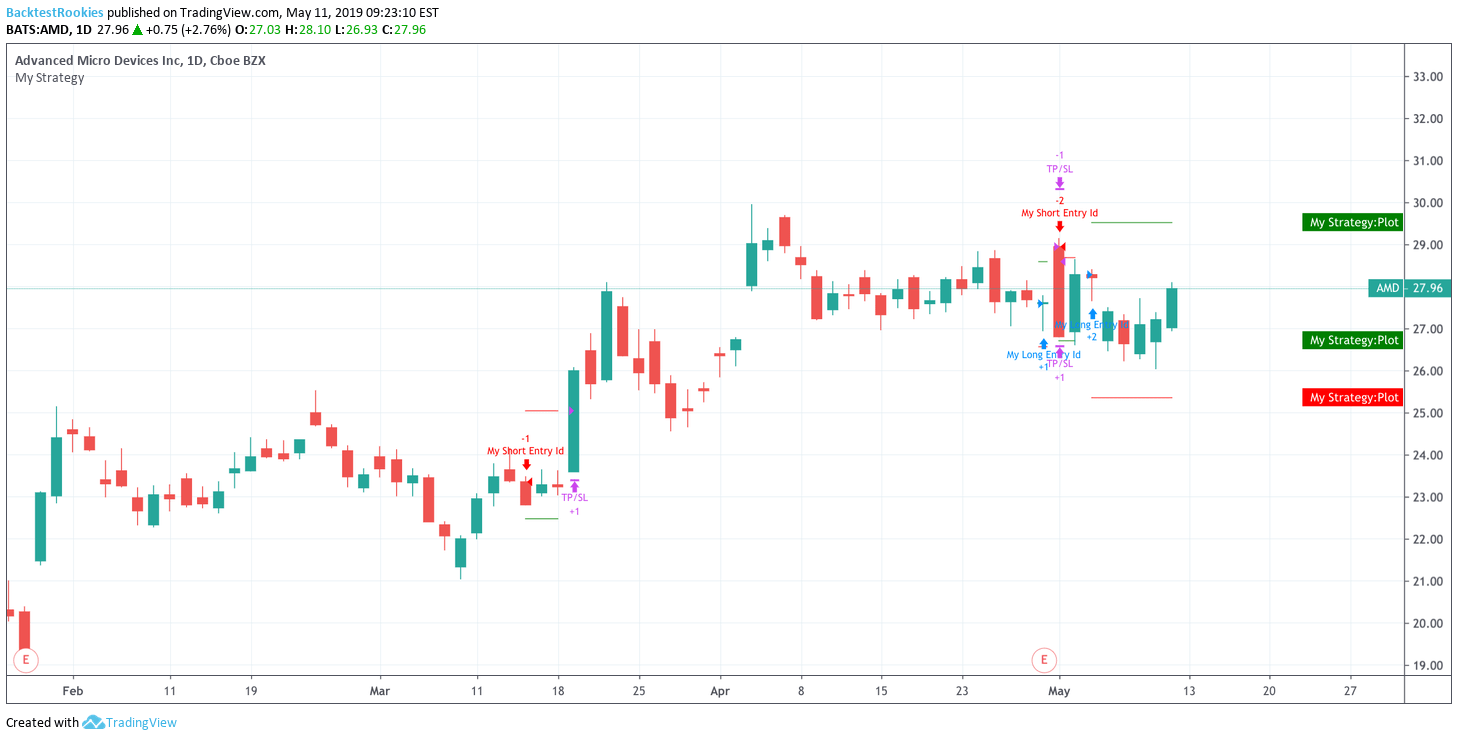## A Simple Alternative for Fixed % Stops / Profits

If you just simply want to set a stop loss or take profit at x% distance away from your entry level and you are NOT pyramiding (entering in the same direction multiple times), then you can just use the variable `strategy.position_avg_price`to calculate the lines.(Note this example also features in the

`//@version=3strategy("Stop Loss Example: Simple Stoploss", overlay=true)sma_per = input(200, title='SMA Lookback Period', minval=1)sl_inp = input(2.0, title='Stop Loss %', type=float)/100tp_inp = input(4.0, title='Take Profit %', type=float)/100sma = sma(close, sma_per)stop_level = strategy.position_avg_price * (1 - sl_inp)take_level = strategy.position_avg_price * (1 + tp_inp)strategy.entry("Simple SMA Entry", strategy.long, when=crossover(close, sma))strategy.exit("Stop Loss/TP","Simple SMA Entry", stop=stop_level, limit=take_level)plot(sma, color=orange, linewidth=2)plot(stop_level, color=red, style=linebr, linewidth=2)plot(take_level, color=green, style=linebr, linewidth=2)`
##### Mini Commentary

When we don’t have a position in the market the `strategy.position_avg_price`value equals `na`. This is helpful for plotting because we are able to plot the stop loss line only when we are in a position. `na`is never plotted and therefore we don’t need to add a ternary conditional operator like in the example above. .Note: To ensure our lines are plotted tidily, the `linebr`style should be used. This will ensure that you don’t have long lines joining up gaps between when the value is `na`. You will see the difference if you just use a normal line style.